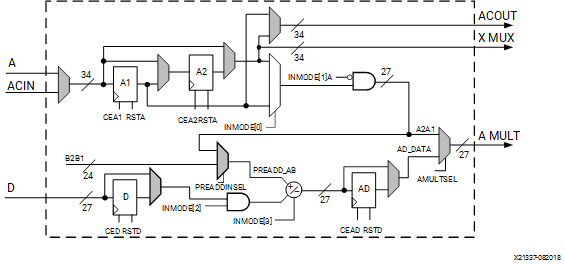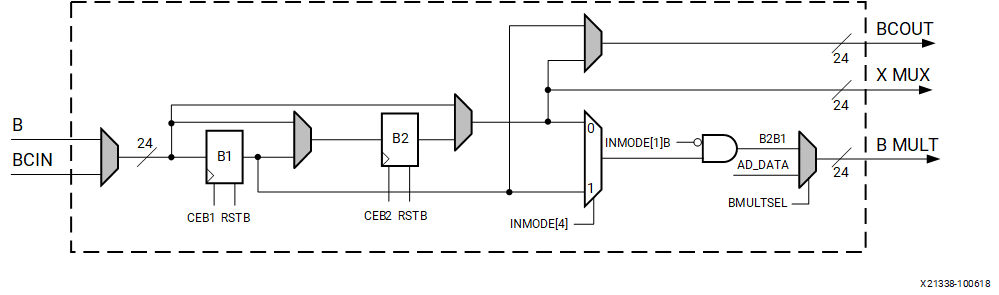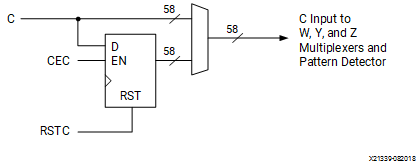# A, B, C, and D Ports

## Versal ACAP DSP Engine Architecture Manual (AM004)

Document ID
AM004
Release Date
2022-09-11
Revision
1.2.1 English

The DSP58 input data ports support many common algorithms. DSP58 has four direct input data ports labeled A, B, C, and D. The A data port is 34 bits wide, the B data port is 24 bits wide, the C data port is 58 bits wide, and the pre-adder D data port is 27 bits wide.

The 27-bit A (A[26:0]) and 24-bit B ports supply input data to the 27-bit by 24-bit, two’s complement multiplier. With the independent C port, each DSP58 is capable of multiply-add, multiply-subtract, and multiply-round operations.

Concatenated A and B ports (A:B) bypass the multiplier and feed the X multiplexer input. The 34-bit A input port forms the upper 34 bits of A:B concatenated datapath, and the 24-bit B input port forms the lower 24 bits of the A:B datapath. The A:B datapath, together with the C input port, enables each DSP58 to implement a full 58-bit adder/subtracter provided the multiplier is not used, which is achieved by setting USE_MULT to NONE.

Each DSP58 also has two cascaded input datapaths (ACIN and BCIN) that provide a cascaded input stream between adjacent DSP58s in the same column. The cascaded path is 34 bits wide for the A input and 24 bits wide for the B input. Applications benefiting from this feature include FIR filters, complex multiplication (larger than 18 × 18), multi-precision multiplication, and complex MACCs (larger than 18 × 18).

The A and B input port and the ACIN and BCIN cascade port can have 0, 1, or 2 pipeline stages in its datapath. The dual A, D, and pre-adder port logic is shown in Figure 1. The dual B register port logic is shown in Figure 2. The different pipestages are set using attributes. Attributes AREG and BREG are used to select the number of pipeline stages for A and B direct inputs to the X multiplexer to the ALU, and INMODE can dynamically change the number of pipeline stages to the multiplier. Attributes ACASCREG and BCASCREG select the number of pipeline stages in the ACOUT and BCOUT cascade datapaths. The allowed attribute settings are shown in Table 1. Multiplexers controlled by configuration bits select flow through paths, optional registers, or cascaded inputs. The data port registers allow users to typically trade off increased clock frequency (that is, higher performance) versus data latency.

Figure 1. Dual A, D, and Pre-Adder LogicFigure 2. Dual B Register LogicThe following table shows the encoding for the INMODE[4:0] dynamic control bits and AMULTSEL, BMULTSEL, and PREADDINSEL static control bits.

These bits select the functionality of the pre-adder, the A, B, and D input registers. AMULTSEL and/or BMULTSEL must be set to AD to enable the pre-adder functions described in the table. Additionally, a new dynamic control port called NEGATE, is used to conditionally negate the multiplier product.

In summary, the INMODE dynamic control signals along with AMULTSEL, BMULTSEL, and PREADDINSEL static attributes control the pre-adder functionality and A, B, and D register bus multiplexers that precede the multiplier, as well as the multiplier product negation controlled by NEGATE control signals that have an effect on the multiplier. The DSP58 supports two-deep A or B sourcing the pre-adder as well as a pre-adder squaring function.

Table 1. INMODE[4:0] Functions with Pre-Adder Options
NEGATE 4 INMODE INMODE INMODE INMODE INMODEA 1 INMODEB 1 INMODE PREADDINSEL BMULTSEL AMULTSEL Multiplier A Port Multiplier B Port 3 Pre-Adder/Multiplier Function
0/1 0/1 0 0 0 0 0 0/1 A/B B A A2/A1 B2/B1 ±A[26:0] * B[23:0]
0/1 0/1 0/1 1 0 0 0 0/1 A B AD D ± A2/A1 2 B2/B1 ±(D[25:0] ± A[25:0]) * B[23:0]
0/1 0/1 0 0 1 1 0 X A B A Zero B2/B1 Zero
0/1 0/1 0 0 1 0 1 X B B A A2/A1 Zero Zero
0/1 X 0/1 1 0 0 0 0/1 A AD AD D ± A2/A1 2 D ± A2/A1 2 ±(D[22:0] ± A[22:0]) 2
0/1 X 0 1 1 1 0 X A AD AD D D ±D[23:0] 2
0/1 X 0/1 0 0 0 0 0/1 A AD AD ± A2/A1 ± A2/A1 ±A[23:0] 2
0/1 X 0/1 1 0 0 0 0/1 A AD A A2/A1 D ± A2/A1 2 ±(D[22:0] ± A[22:0]) * A[22:0]
0/1 0/1 0/1 1 0 0 0 0/1 B AD A A2/A1 D ± B2/B1 2 ±(D[22:0] ± B[22:0]) * A[26:0]
0/1 X 0 1 1 0 1 0/1 B AD A A2/A1 D ±D[23:0] * A[26:0]
0/1 0/1 0/1 1 0 0 0 0/1 B AD AD D ± B2/B1 2 D ± B2/B1 2 ±(D[22:0] ± B[22:0]) 2
0/1 0/1 0/1 0 0 0 0 0/1 B AD AD ± B2/B1 ± B2/B1 ±B[23:0] 2
0/1 0/1 0/1 1 0 0 0 0/1 B B AD D ± B2/B1 2 B2/B1 ±(D[25:0] ± B[22:0]) * B[22:0]
A 0 X 0 0 0 0 0 X B A A B = 1 |A[26:0]|
B 0 X 0 0 0 0 0 X B A A = 1 B |B[23:0]|
D 0/1 0 1 1 1 0 0/1 A B AD D + Zerp B = 1 |D[26:0]|
1. INMODEA and INMODEB are internal signals defined by the user settings of PREADDINSEL and INMODE. If PREADDINSEL=A, INMODEA (see Figure 1) is INMODE and INMODEB (see Figure 2) is 0. If PREADDINSEL=B, INMODEB is INMODE and INMODEA is 0.
2. Set the data on the D and the A or B ports so the pre-adder, which does not support saturation, does not overflow or underflow. See Pre-Adder.
3. A or D are limited to 24-bit (if only one bus is 0) when provided through the B port (if the pre-adder is configured as a multiplexer). A or D are limited to 23-bit two's complement signed extended numbers when both are non-zero, PREADDINSEL = A, BMULTSEL = AD, and the pre-adder is configured as an adder/subtracter.
4. With DSP58, set NEGATE = 1 to negate the product instead of using the pre-adder to negate one of the inputs. Doing so skips the pre-adder stage, which at high clock frequencies, reduces one cycle of latency. Furthermore, the pre-adder can only support 26-bit two’s complement negation whereas the NEGATE pin directly negates the product of the 27 × 24 multiplier.

INMODE selects between A1 (INMODE = 1) and the A2 MUX controlled by AREG (INMODE = 0).

INMODE can be used to gate the A or B datapath to use the pre-adder to create a 2:1 bus multiplexer along with the INMODE control signal.

When INMODE = 0, the D input to the pre-adder is 0. INMODE and INMODE enable multiplexing between the D register and the A or B registers, without having to use resets to force them to zero. For information on how to configure the preadder as a 2:1 multiplexer, see Pre-Adder Block Applications.

INMODE provides pre-adder subtract control, where INMODE = 1 indicates subtract and INMODE = 0 indicates add of A or B to D. When D is gated off, this dynamic inversion can provide the absolute value of A or B.

INMODE selects between B1 (INMODE = 1) and the B2 MUX controlled by BREG (INMODE = 0).

The 58-bit C port is used as a general input to the W, Y, and Z multiplexers to perform add, subtract, four-input add/subtract, and logic functions. The C input is also connected to the pattern detector and can be used to support rounding function implementations. The C port logic is shown in the following figure. The CREG attribute selects the number of pipestages for the C input datapath.

Figure 3. C Port Logic